Importance: Low ✭
 Author(s): Brewster, Richard C. Noel, Jonathan A.
 Subject: Graph Theory » Coloring » » Vertex coloring
 Keywords: discrete homotopy graph colourings mixing
 Posted by: Jon Noel on: September 22nd, 2012
Question   Isalways rational?

Given a proper-colouringof a graph, consider the following 'mixing process:'

\item choose a vertex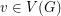; \item change the colour of(if possible) to yield a different-colouringof.

A natural problem arises: Can every-colouring ofbe generated fromby repeatedly applying this process? If so, we say thatis-mixing.

The problem of determining if a graph is-mixing and several related problems have been studied in a series of recent papers [1,2,4-6]. The authors of  provide examples which show that a graph can be-mixing but not-mixing for integers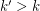. For example, givenconsider the bipartite graphwhich is obtained by deleting a perfect matching from. It is an easy exercise to show that foris-mixing if and only if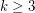and. This example motivates the following definition.

Definition   Define the mixing threshold ofto beAn analogous definition can be made for circular colouring. Recall, a-colouring of a graph is a mapping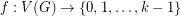such that if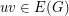, then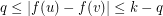. As with ordinary colourings, we say that a graphis-mixing if all-colourings ofcan be generated from a single-colouringby recolouring one vertex at a time.

Definition   Define the circular mixing threshold ofto be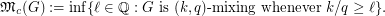Several bounds on the circular mixing threshold are obtained in , including the following which relates the circular mixing threshold to the mixing threshold.

Theorem  (Brewster and Noel] For every graph,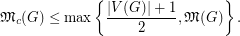As a corollary, we have the following: if, then. However, examples in  show that the ratio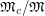can be arbitrarily large in general.

Regarding the problem of determining ifis rational, it is worth mentioning that there are no known examples of graphsfor whichis not an integer.

Other problems are also given in . One can check that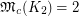and. However, the only graphs which are known to satisfy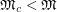are in some sense related to, eg. trees and complete bipartite graphs.

Question   Is there a non-bipartite graphsuch that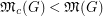?

Using an example from , it is shown in  that ifis an integer, then there is a graphsuch thatif and only if. A natural question to ask is whether a similar result holds for the circular chromatic number. Again, certain bipartite graphs are an exception.

Question   Is there a non-bipartite graphsuch that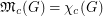?

Also, the example ofshows that the circular mixing threshold is, in general, not attained. However, the following problem is open.

Question   Is the circular mixing threshold always attained for non-bipartite graphs?

For more precise versions of the last three questions, see .

## Bibliography

 P. Bonsma and L. Cereceda. Finding paths between graph colourings: PSPACE-completeness and superpolynomial distances. Theoret. Comput. Sci. 410 (2009), (50): 5215--5226.

 P. Bonsma, L. Cereceda, J. van den Heuvel, and M. Johnson. Finding paths between graph colourings: Computational complexity and possible distances. Electronic Notes in Discrete Mathematics 29 (2007): 463--469.

* R. C. Brewster and J. A. Noel. Mixing Homomorphisms and Extending Circular Colourings. Submitted. pdf.

 L. Cereceda, J. van den Heuvel, and M. Johnson. Connectedness of the graph of vertex-colourings. Discrete Math. 308 (2008), (5-6): 913--919.

 L. Cereceda, J. van den Heuvel, and M. Johnson. Mixing 3-colourings in bipartite graphs. European J. Combin. 30 (2009), (7): 1593--1606.

 L. Cereceda, J. van den Heuvel, and M. Johnson. Finding paths between 3-colorings. Journal of Graph Theory, 67 (2011), (1): 69--82.

 J. A. Noel. "Jonathan Noel - Mixing Circular Colourings." Webpage.

* indicates original appearance(s) of problem.